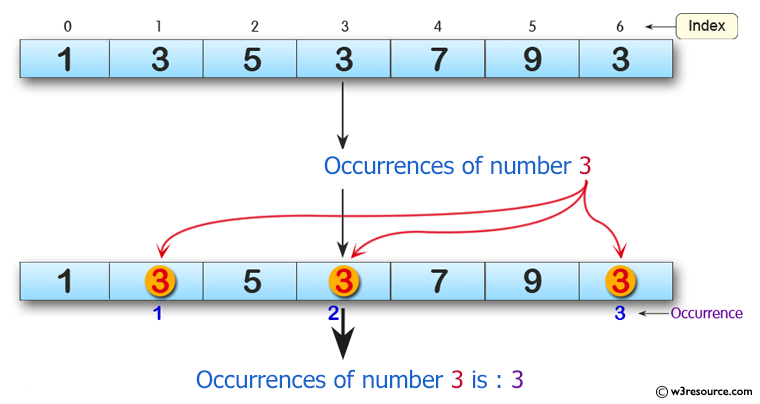﻿ Python: Get the number of occurrences of a specified element in an array - w3resource# Python: Get the number of occurrences of a specified element in an array

## Python: Array Exercise-6 with Solution

Write a Python program to get the number of occurrences of a specified element in an array.

Pictorial Presentation:Sample Solution:

Python Code :

``````from array import *
array_num = array('i', [1, 3, 5, 3, 7, 9, 3])
print("Original array: "+str(array_num))
print("Number of occurrences of the number 3 in the said array: "+str(array_num.count(3)))
``````

Sample Output:

```Original array: array('i', [1, 3, 5, 3, 7, 9, 3])
Number of occurrences of the number 3 in the said array: 3
```

Python Code Editor:

What is the difficulty level of this exercise?

Test your Python skills with w3resource's quiz

﻿

## Python: Tips of the Day

Python: Unpack a Tuple

```>>> items = (0, 'b', 'one', 10,  11, 'zero')
>>> a, b, c, d, e, f = items
>>> print(f)
zero
>>> a, *b, c = items
>>> print(b)
['b', 'one', 10, 11]
>>> *_, a, b = items
>>> print(a)
11
```